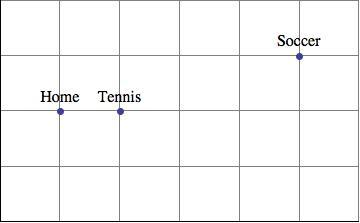# Equations and Inequalities

Lesson 7

Math

Unit 6

Lesson 7 of 14

## Objective

Solve multi-part equations leading to the form ${x+p=q }$  and ${px=q}$.

## Common Core Standards

### Core Standards

• 6.EE.B.6 — Use variables to represent numbers and write expressions when solving a real-world or mathematical problem; understand that a variable can represent an unknown number, or, depending on the purpose at hand, any number in a specified set.
• 6.EE.B.7 — Solve real-world and mathematical problems by writing and solving equations of the form x + p = q and px = q for cases in which p, q and x are all nonnegative rational numbers.

• 6.EE.A.3

## Criteria for Success

1. Use diagrams to represent multi-part real-world situations.
2. Write algebraic expressions to represent information in diagrams.
3. Write and solve equations in the form ${ x+p=q }$ and ${px=q}$ from real-world problems.

## Tips for Teachers

• The problems in this lesson all involve more than one step; however, the resulting equations will always be in the form $x+p=q$ or $px=q$. Students can employ different strategies to make sense of the problem and map out their solution pathway, such as drawing diagrams, simplifying the problem, explaining the problem in their own words, or listening to a peer explain it in his or her words, etc. (MP.1).
• In this lesson, students again engage in MP.2. They use symbols to represent situations, defining their own variables, and then interpret their numerical solutions in context to understand what it tells them about the problem.
Fishtank Plus

Unlock features to optimize your prep time, plan engaging lessons, and monitor student progress.

## Anchor Problems

### Problem 1

The Jonas family had a really busy day. After leaving their home, Mrs. Jonas dropped son Cody at his tennis practice. She then drove daughter Kristin to her soccer game and stayed to watch. After the game, mother and daughter picked up Cody on the way home. Once home, Mrs. Jonas saw that they had driven 20 miles that day.

The map below shows the locations of where the Jonas family traveled. The car can only travel along the main streets (gridlines), and all distances between cross streets are the same distance. Assume Mrs. Jonas took the most direct routes to and from her destinations.How far are the tennis courts from home?

Set up and solve an equation in one variable to find the distance between the tennis courts and home.

#### References

Illustrative Mathematics Busy Day

Busy Day, accessed on Feb. 28, 2018, 2:32 p.m., is licensed by Illustrative Mathematics under either the CC BY 4.0 or CC BY-NC-SA 4.0. For further information, contact Illustrative Mathematics.

Modified by Fishtank Learning, Inc.

### Problem 2

Sierra walks her dog Pepper twice a day. Her evening walk is two-and-a-half times as far as her morning walk. At the end of the week she tells her mom, “I walked Pepper for 30 miles this week!”

How long is her morning walk? Write and solve an equation.

#### References

Illustrative Mathematics Morning Walk

Morning Walk, accessed on Feb. 28, 2018, 2:33 p.m., is licensed by Illustrative Mathematics under either the CC BY 4.0 or CC BY-NC-SA 4.0. For further information, contact Illustrative Mathematics.

Modified by Fishtank Learning, Inc.

### Problem 3

The school librarian, Mr. Marker, knows the library has 1,400 books, but he wants to reorganize how the books are displayed on the shelves. Mr. Marker needs to know how many fiction, nonfiction, and resource books are in the library. He knows that:

• There are four times as many fiction books as resource books.
• There are half as many nonfiction books as fiction books.

If these are the only types of books in the library, how many of each type of book are in the library?
Draw a tape diagram to represent the books in the library, and then write and solve an equation to determine how many of each type of book there are in the library.

#### References

EngageNY Mathematics Grade 6 Mathematics > Module 4 > Topic G > Lesson 29Example

Grade 6 Mathematics > Module 4 > Topic G > Lesson 29 of the New York State Common Core Mathematics Curriculum from EngageNY and Great Minds. © 2015 Great Minds. Licensed by EngageNY of the New York State Education Department under the CC BY-NC-SA 3.0 US license. Accessed Dec. 2, 2016, 5:15 p.m..

Modified by Fishtank Learning, Inc.

## Problem Set

The following resources include problems and activities aligned to the objective of the lesson that can be used to create your own problem set.

• Include problems where students solve one-step equations without context to ensure students get procedural practice.
• Include problems where students solve one-step equations without context to ensure students get procedural practice.

A town's total allocation for firefighters’ wages and beneﬁts in a new budget is $600,000. If wages are calculated at$40,000 per firefighter and beneﬁts at \$20,000 per firefighter, write an equation whose solution is the number of firefighters the town can employ if they spend their whole budget. Solve the equation.

#### References

Illustrative Mathematics Firefighter Allocation

Firefighter Allocation, accessed on Nov. 6, 2017, 9:04 a.m., is licensed by Illustrative Mathematics under either the CC BY 4.0 or CC BY-NC-SA 4.0. For further information, contact Illustrative Mathematics.

Lesson 6

Lesson 8

## Lesson Map

Topic A: Reasoning About and Solving Equations

Topic B: Reasoning About and Solving Inequalities

Topic C: Representing and Analyzing Quantitative Relationships